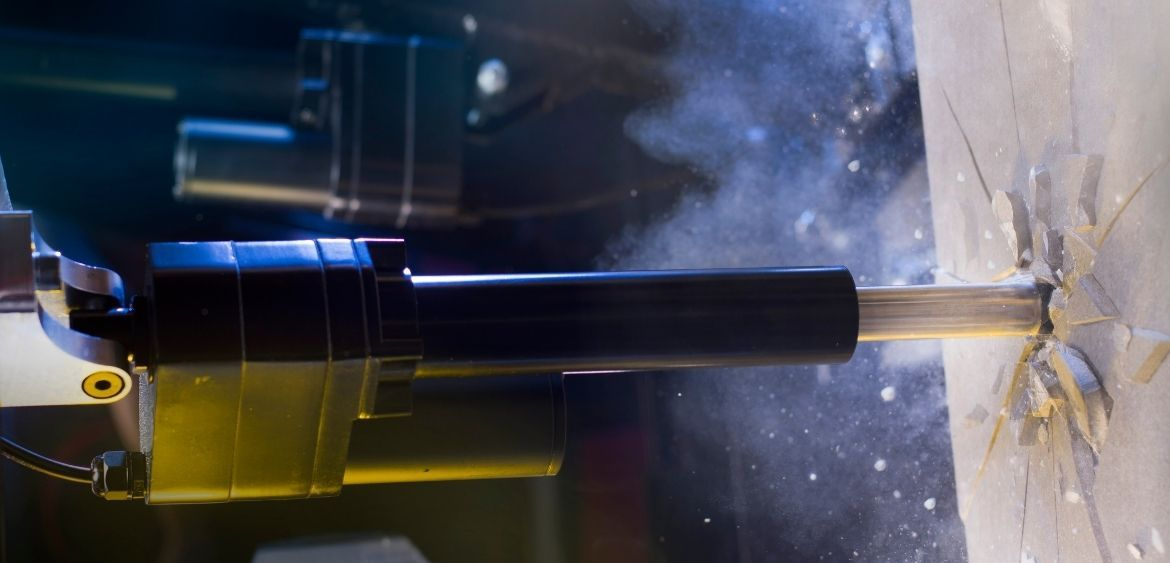# Linear Actuators: Calculating Stroke Length And ForceAnna Sapiga
PA Engineer

The first step in determining the size of a linear actuator needed to move a specific load requires the user to calculate translational force. To calculate the translational force, four components must be considered: mass, friction, gravity, and other counter-forces within the system. Each component must be solved for in the equation to yield the appropriately sized actuator. A sample calculation will be provided to help users determine the size of the actuator. A list of terms related to the calculation is also necessary from a proper understanding of the variables in the equations.

Browse through our range of actuators to find your perfect match

## Variables in the Linear Actuator Equation

The terms and variables related to determining the size of linear actuators are explained below to help you understand the equation that follows:

T = Total Linear Force (lbf)
Ff = Force From Friction (lbf)
Fa = Acceleration Force (lbf)
Fg = Force Due to Gravity (lbf)
Fp = Applied Force (lbf)
WL = Weight of Load (lbf)
U = Angle of Inclination (lbf)
ta = acceleration time (sec)
v = final velocity
µ = coefficient of sliding friction
g = acceleration of gravity = 386.4 in/sec.

## Sample Calculation for Actuator Selection

In the sample calculation, users will determine the force or thrust required to accelerate a 200-pound mass eight inches. This mass should be moved within two seconds. In the example, users should assume the load is on an incline at 30 degrees. The sliding coefficient of friction is 0.15. For the sake of fully demonstrating how this equation works, assume there is a 25-pound spring force located in the opposite direction of motion.

The first step in determining the size of actuators is to calculate total force. This can be accomplished by summing up all the forces. These forces include friction, acceleration, gravity and applied force. The equation is as follows:

T = Ff + Fa + Fg + Fp

The next phase is to actually employ the use of the total force equation. The abstract form of the equation is as follows:

T = WLµ cos (??) + (WL/386.4) * (v/ta) + WL sin (??) + Fp

The final and last phase is to insert the numbers from the example. In this instance, the variables consist of the following:

WL = 200 pounds
µ = 0.15
?? = 30°
cos (??) = 0.866
v = 8 inches
ta = 0.2 (2 seconds)
sin (??) = 0.5
Fp = 25 pounds

When the numbers are inserted into the equation properly, the final equation looks like this:

T = 200 * 0.15 * 0.866 + (200/386.4) * ( 8/0.2) + 200 * 0.5 + 25

The final calculation yields a force equal to the following:

= 26 + 20.73 + 100 + 25

= 171.73 lbf

Use our Actuator Compare Tool to check and compare multiple actuators at once!

## Select The Appropriate Automation Device

Now that the force required for this application has been calculated, the proper actuator can be selected. In this instance, actuators with a rating of up to 200 pounds would suffice. Standard linear actuators would be required. Typically, standard linear actuators can withstand forces between 150 and 400 pounds.